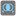• # Returning null

## Question related to missionBoolean Algebra

Hi, I am new to programming and a little embarrassed my code is so cumbersome here, but after testing my function it is returning null. The feedback also says boolean(0, 0, u"conjunction"). Is this what it is testing as arguments to my function? The u before "conjunction" threw me off. Maybe it is a mistake on my part though. Anyways any help would be greatly appreciated.

My code:

```def boolean(x, y, operation):
if operation is "conjunction":
if x is 0 and y is 0:
return 0
elif x is 0 and y is 1:
return 0
elif x is 1 and y is 0:
return 0
elif x is 1 and y is 1:
return 1
elif operation is "disjunction":
if x is 0 and y is 0:
return 0
elif x is 0 and y is 1:
return 1
elif x is 1 and y is 0:
return 1
elif x is 1 and y is 1:
return 1
elif operation is "implication":
if x is 0 and y is 0:
return 1
elif x is 0 and y is 1:
return 1
elif x is 1 and y is 0:
return 0
elif x is 1 and y is 1:
return 1
elif operation is "exclusive":
if x is 0 and y is 0:
return 0
elif x is 0 and y is 1:
return 1
elif x is 1 and y is 0:
return 1
elif x is 1 and y is 1:
return 0
elif operation is "equivalence":
if x is 0 and y is 0:
return 1
elif x is 0 and y is 1:
return 0
elif x is 1 and y is 0:
return 0
elif x is 1 and y is 1:
return 1
```5